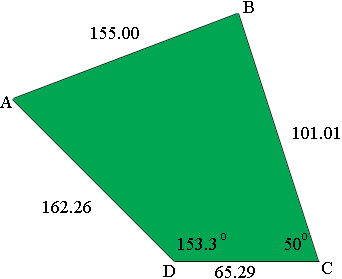SEARCH HOMEMath Central Quandaries & QueriesQuestion from michelle: the front is 65.29 left side 162.26 the back 155.00 right side 101.01 right angle=50.00 left angle= 153.30 Thank You! MichelleMichelle,

Before I do the calculations I want to make sure I have the diagram correct. The diagram is not to scale and I didn't measure the angles I just want to know if I have the angles you gave between the correct sides.Write back and let me know.
Harley

Michelle wrote back

Yes that is what it looks like. It's a culdasac. Thanks! Michelle

Now that I am certain I have the angles in the correct places I can use our size caculator for four-sided lots. I labeled the verticies clockwise as instructed and input the lengths of the four sides and the angle at C as 50o. The result was 0.19 acres. I then redid the calculations with the angle at D as 153.3o. The result was 0.21 acres.

I expected closer agreement. The best I can say is that the area is 0.2 acres.

HarleyMath Central is supported by the University of Regina and The Pacific Institute for the Mathematical Sciences.